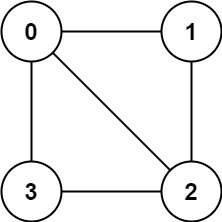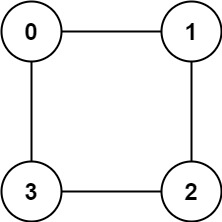# 785. Is Graph Bipartite? ⭐

Medium
There is an undirected graph with `n` nodes, where each node is numbered between `0` and `n - 1`. You are given a 2D array `graph`, where `graph[u]` is an array of nodes that node `u` is adjacent to. More formally, for each `v` in `graph[u]`, there is an undirected edge between node `u` and node `v`. The graph has the following properties:
• There are no self-edges (`graph[u]` does not contain `u`).
• There are no parallel edges (`graph[u]` does not contain duplicate values).
• If `v` is in `graph[u]`, then `u` is in `graph[v]` (the graph is undirected).
• The graph may not be connected, meaning there may be two nodes `u` and `v` such that there is no path between them.
A graph is bipartite if the nodes can be partitioned into two independent sets `A` and `B` such that every edge in the graph connects a node in set `A` and a node in set `B`.
Return `true` if and only if it is bipartite.
Example 1:Input: graph = [[1,2,3],[0,2],[0,1,3],[0,2]]
Output: false
Explanation: There is no way to partition the nodes into two independent sets such that every edge connects a node in one and a node in the other.
Example 2:Input: graph = [[1,3],[0,2],[1,3],[0,2]]
Output: true
Explanation: We can partition the nodes into two sets: {0, 2} and {1, 3}.
Constraints:
• `graph.length == n`
• `1 <= n <= 100`
• `0 <= graph[u].length < n`
• `0 <= graph[u][i] <= n - 1`
• `graph[u]` does not contain `u`.
• All the values of `graph[u]` are unique.
• If `graph[u]` contains `v`, then `graph[v]` contains `u`.

### 解題

func isBipartite(graph [][]int) bool {
// 著色，相鄰的點應該不同色
arr := make([]int, len(graph))
var dfs func(int, int) bool // 用來遍歷連通的點進行著色
dfs = func(n, color int) bool {
arr[n] = color
for _, node := range graph[n] {
if arr[node] == color {
return false
}
if arr[node] == 0 && !dfs(node, -color) {
return false
}
}
return true
}
for i := 0; i < len(arr); i++ {
if arr[i] == 0 {
if !dfs(i, 1) {
return false
}
}
}
return true
}